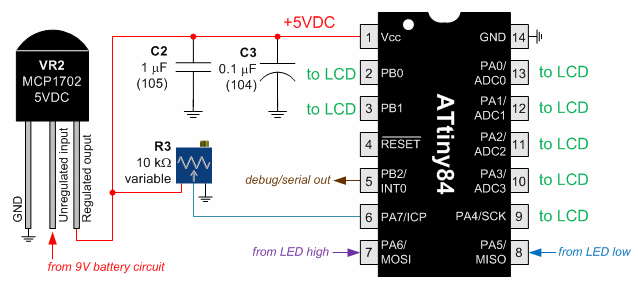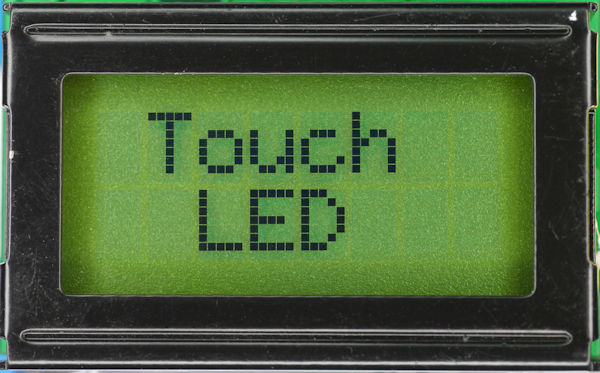• Home• Patreon• Links• Books
LED Tester with Display:

# LED Tester Microcontroller

On the previous page, the LED is current limited by an LM317 circuit. Additionally, two voltage measurement points are brought out for determining the LED voltage drop and current.

The microcontroller side of the circuit is just as simple as the current limiter.LED Tester microcontroller schematic

VR2: This regulates voltage, instead of current. It produces a steady 5V power source for the microcontroller and LCD.

C2, C3: These capacitors store the 5V power to keep things steady as the microcontroller and LCD vary their power demands.

R3: 10 kilohm trimpot. This trimpot does nothing more than provide a method for the user to tell the microcontroller the target voltage to use for the resistor calculation. That is, the position of the potentiometer wiper only changes the voltage at input pin 6 (PA7) from 5V to 0V. The potentiometer does not actually control the voltage to the LED or anything else. The microcontroller arbitrarily scales the input voltage to a value between 9.9V (maximum space on display) and 0V.

Atmel ATtiny84 The microcontroller performs all of the measurements and calculations, and updates the display. As you can see, most of the pins are used for the LCD. (I wrote an article on how a Hitachi display is controlled.)

This microcontroller has 8 KB of memory, although the program takes less than 4 KB. When developing, I find it is always better to buy the largest memory part available, and optimize down for production later. That way, you can turn off compiler optimization and add debug statements to first get everything functioning.

## Source Code

Here’s the code to perform the conversations:

``` int targetVoltageDialInput; int ledVoltageInput; int ledCurrentSenseInput; byte targetVoltageTimes10; byte ledVoltageTimes10; byte ledMilliamps; int recommendedResistor; float adcConversion; #define ROUND_UP 0.5 targetVoltageDialInput = CL_ADCGetSingle10Bit(VOLTAGE_DIAL_ADC); #define TARGET_VOLTAGE_MAXIMUM 9.999 #define INPUT_ADC_MAXIMUM 1024 adcConversion = TARGET_VOLTAGE_MAXIMUM / (float) INPUT_ADC_MAXIMUM * targetVoltageDialInput; targetVoltageTimes10 = (byte)(adcConversion * 10 + ROUND_UP); ledCurrentSenseInput = CL_ADCGetSingle10Bit(LED_GROUND_ADC); #define BOARD_VOLTAGE 5 #define LED_CURRENT_SENSE_RESISTOR 47 adcConversion = BOARD_VOLTAGE / (float) INPUT_ADC_MAXIMUM * ledCurrentSenseInput; // Voltage drop of current sense resistor ledMilliamps = (byte)(adcConversion / LED_CURRENT_SENSE_RESISTOR * 1000 + ROUND_UP); ledVoltageInput = CL_ADCGetSingle10Bit(LED_HALF_VOLTAGE_ADC) * 2; /* LED anode input is half voltage to avoid exceeding the microcontroller’s voltage */ ledVoltageInput = ledVoltageInput - ledCurrentSenseInput; /* Subtract the cathode voltage because it is connected to ground through a current sense */ if (ledVoltageInput < 0) {     ledVoltageInput = 0; } adcConversion = BOARD_VOLTAGE / (float) INPUT_ADC_MAXIMUM * ledVoltageInput; ledVoltageTimes10 = (byte)(adcConversion * 10 + ROUND_UP); #define MAXIMUM_RECOMMENDED_RESISTOR 9999 if (ledMilliamps == 0) {     recommendedResistor = MAXIMUM_RECOMMENDED_RESISTOR; } else {     if (targetVoltageTimes10 <= ledVoltageTimes10)     {         recommendedResistor = 0;     }     else     {         recommendedResistor = (int)((targetVoltageTimes10 - ledVoltageTimes10) / (float)ledMilliamps * 100 + ROUND_UP);         if (recommendedResistor > MAXIMUM_RECOMMENDED_RESISTOR)         {             recommendedResistor = MAXIMUM_RECOMMENDED_RESISTOR;         }     } } ```

If the ledVoltageInput is zero, the display tells the user to touch an LED to the pads. The LCD can be purchased from an auction site for less than \$5. Search for '8x2 LCD'.LCD Display Touch LED

Now let’s put the whole thing together onto a printed circuit board.